position_likert(vjust = 1, reverse = FALSE, exclude_fill_values = NULL)

position_likert_count(vjust = 1, reverse = FALSE, exclude_fill_values = NULL)

## Arguments

vjust

Vertical adjustment for geoms that have a position (like points or lines), not a dimension (like bars or areas). Set to 0 to align with the bottom, 0.5 for the middle, and 1 (the default) for the top.

reverse

If TRUE, will reverse the default stacking order. This is useful if you're rotating both the plot and legend.

exclude_fill_values

Vector of values from the variable associated with the fill aesthetic that should not be displayed (but still taken into account for computing proportions)

## Details

position_likert() stacks proportion bars on top of each other and center them around zero (the same number of modalities are displayed on each side). This type of presentation is commonly used to display Likert-type scales. position_likert_count() uses counts instead of proportions.

It is recommended to use position_likert() with stat_prop() and its complete argument (see examples).

See ggplot2::position_stack() and ggplot2::position_fill()

## Examples

library(ggplot2)

ggplot(diamonds) +
aes(y = clarity, fill = cut) +
geom_bar(position = "fill") +
scale_x_continuous(label = scales::label_percent()) +
scale_fill_brewer(palette = "PiYG") +
xlab("proportion")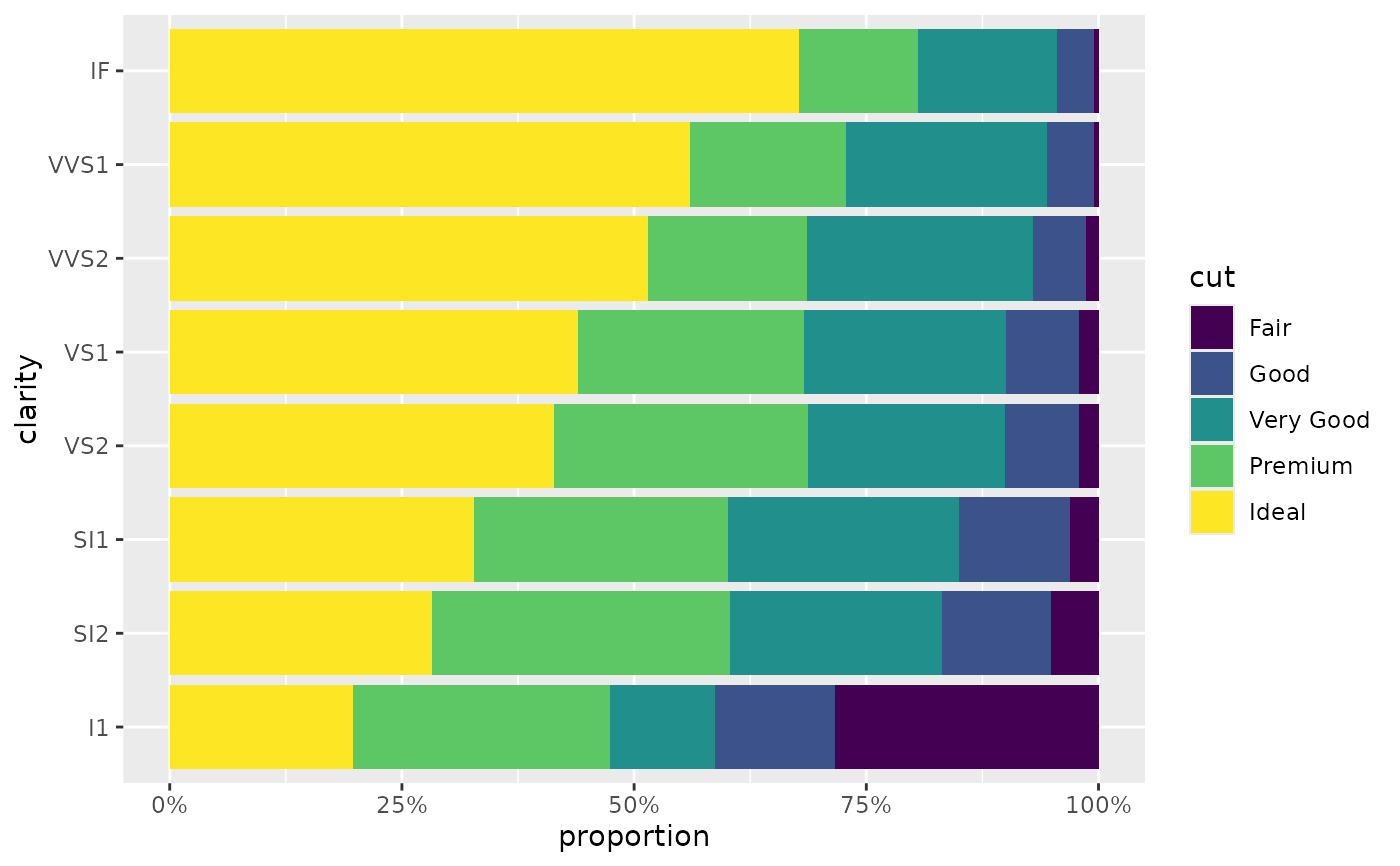ggplot(diamonds) +
aes(y = clarity, fill = cut) +
geom_bar(position = "likert") +
scale_x_continuous(label = label_percent_abs()) +
scale_fill_brewer(palette = "PiYG") +
xlab("proportion")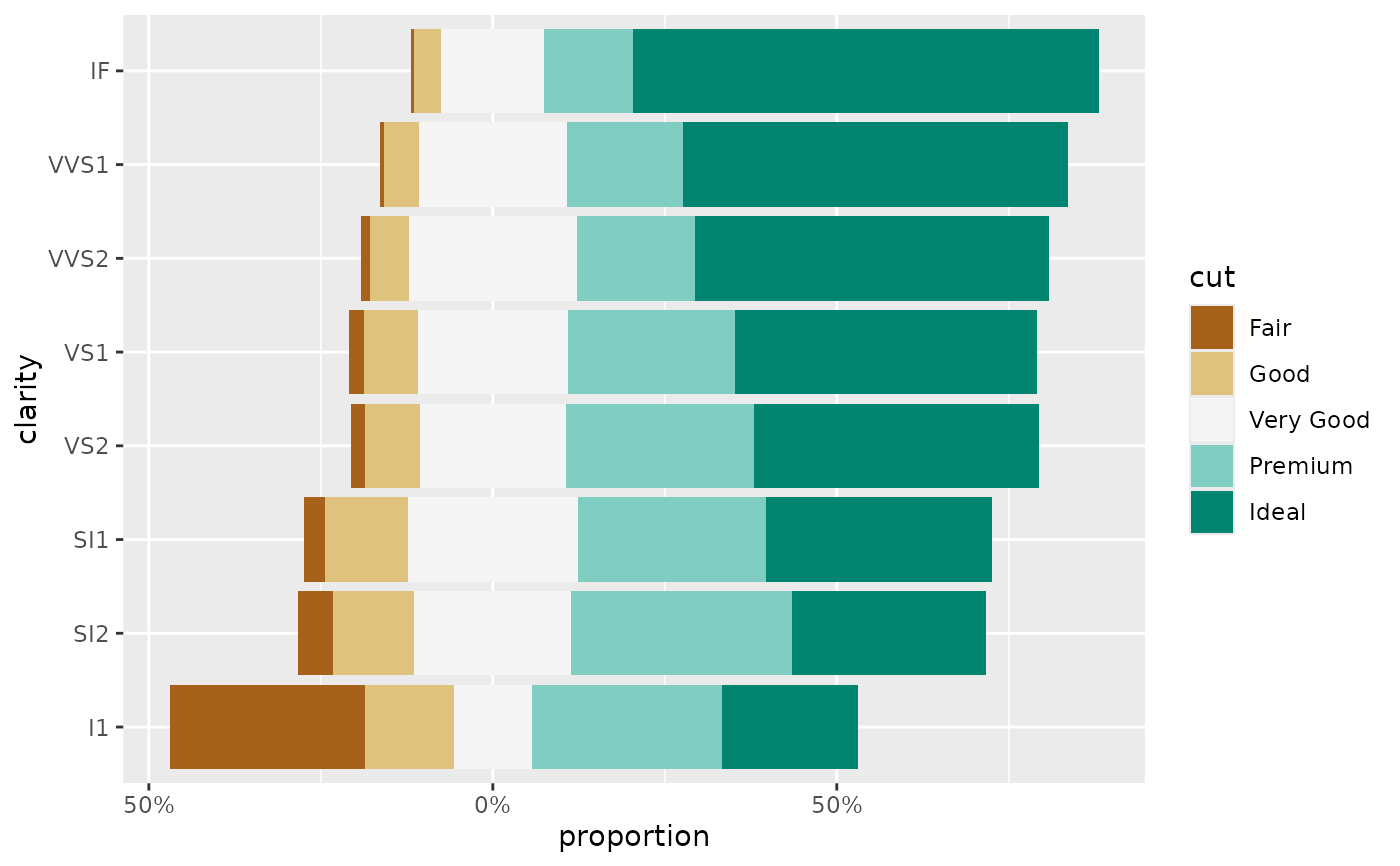ggplot(diamonds) +
aes(y = clarity, fill = cut) +
geom_bar(position = "stack") +
scale_fill_brewer(palette = "PiYG")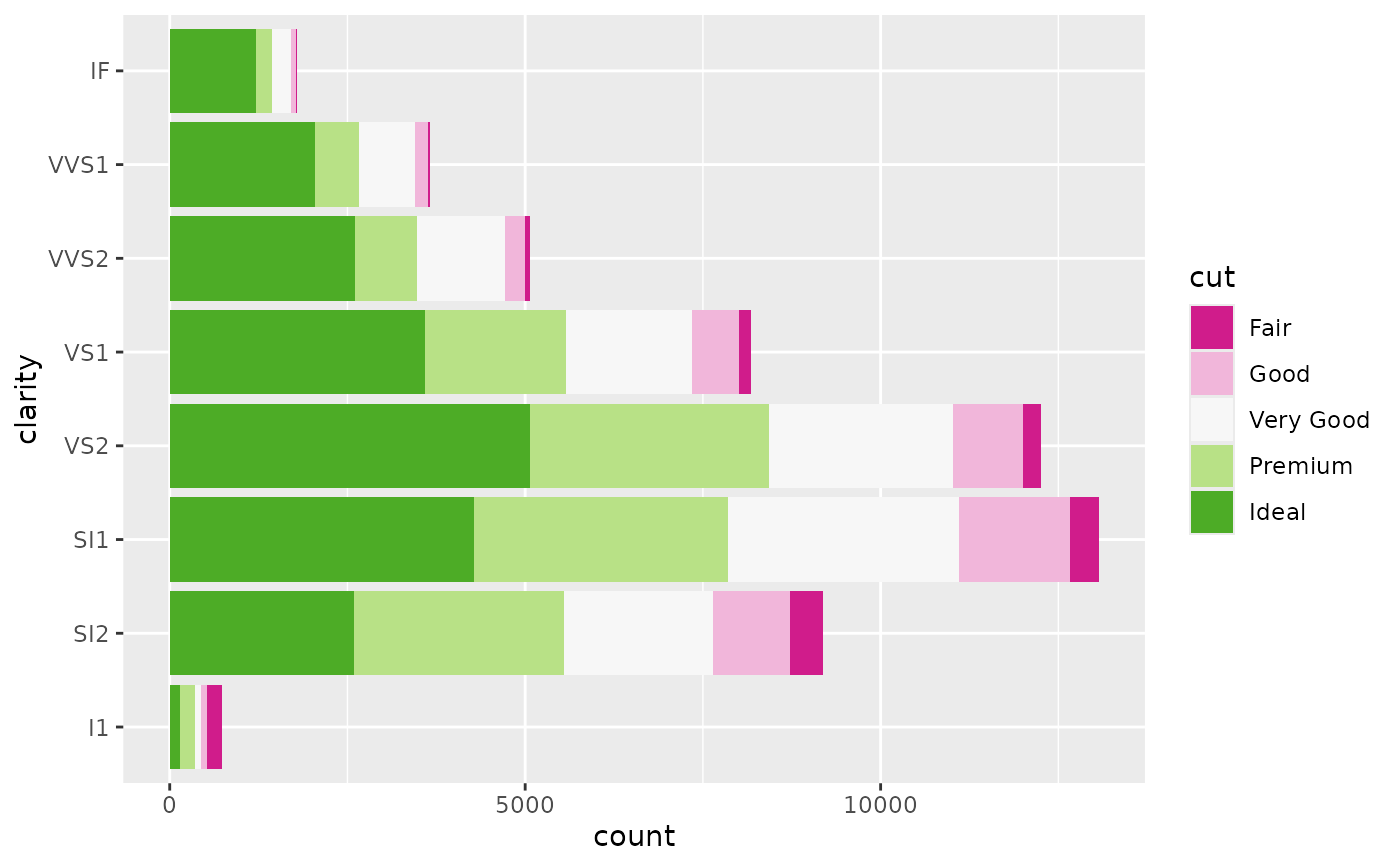ggplot(diamonds) +
aes(y = clarity, fill = cut) +
geom_bar(position = "likert_count") +
scale_x_continuous(label = label_number_abs()) +
scale_fill_brewer(palette = "PiYG")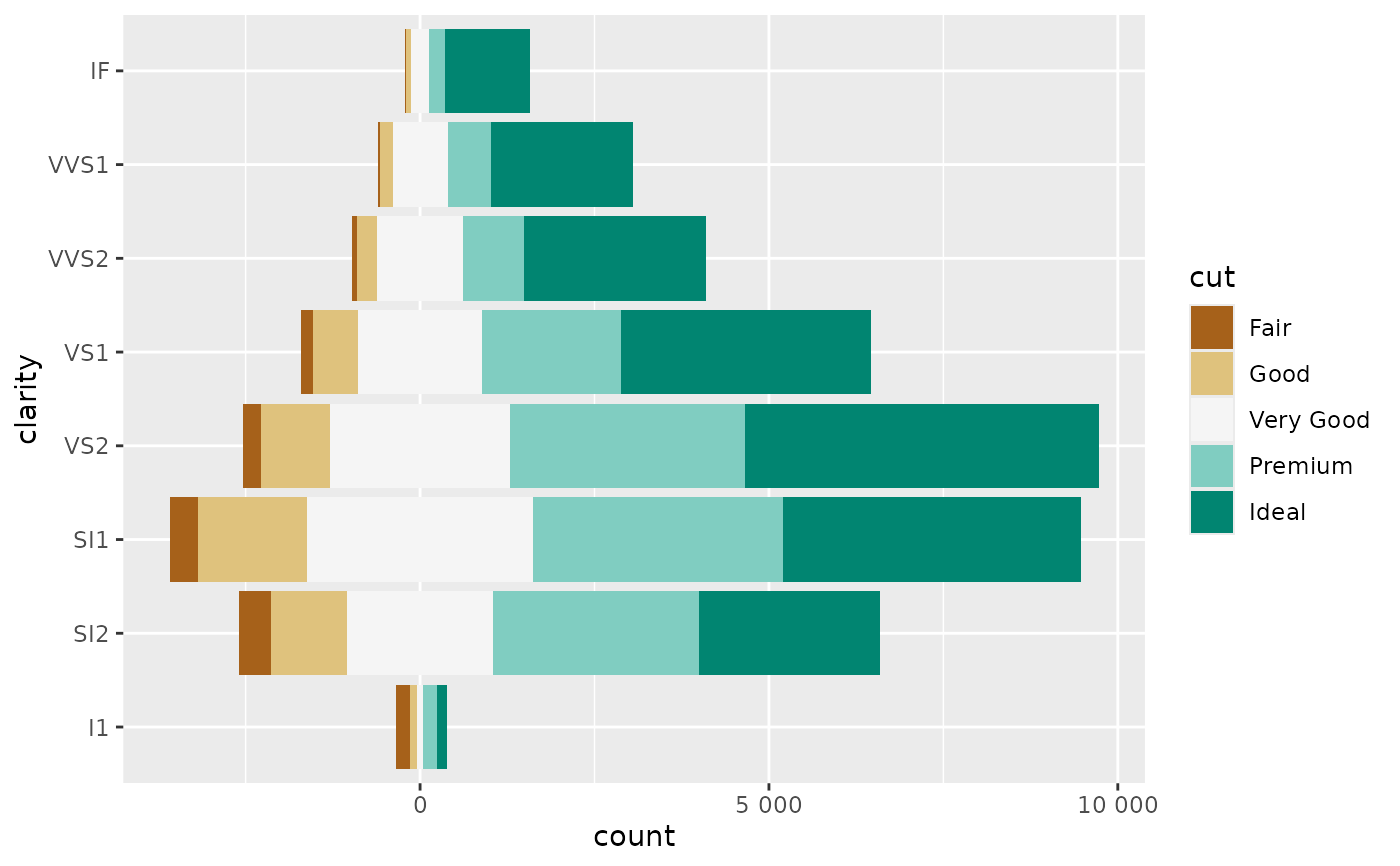# \donttest{
# Reverse order -------------------------------------------------------------

ggplot(diamonds) +
aes(y = clarity, fill = cut) +
geom_bar(position = position_likert(reverse = TRUE)) +
scale_x_continuous(label = label_percent_abs()) +
scale_fill_brewer(palette = "PiYG", direction = -1) +
xlab("proportion")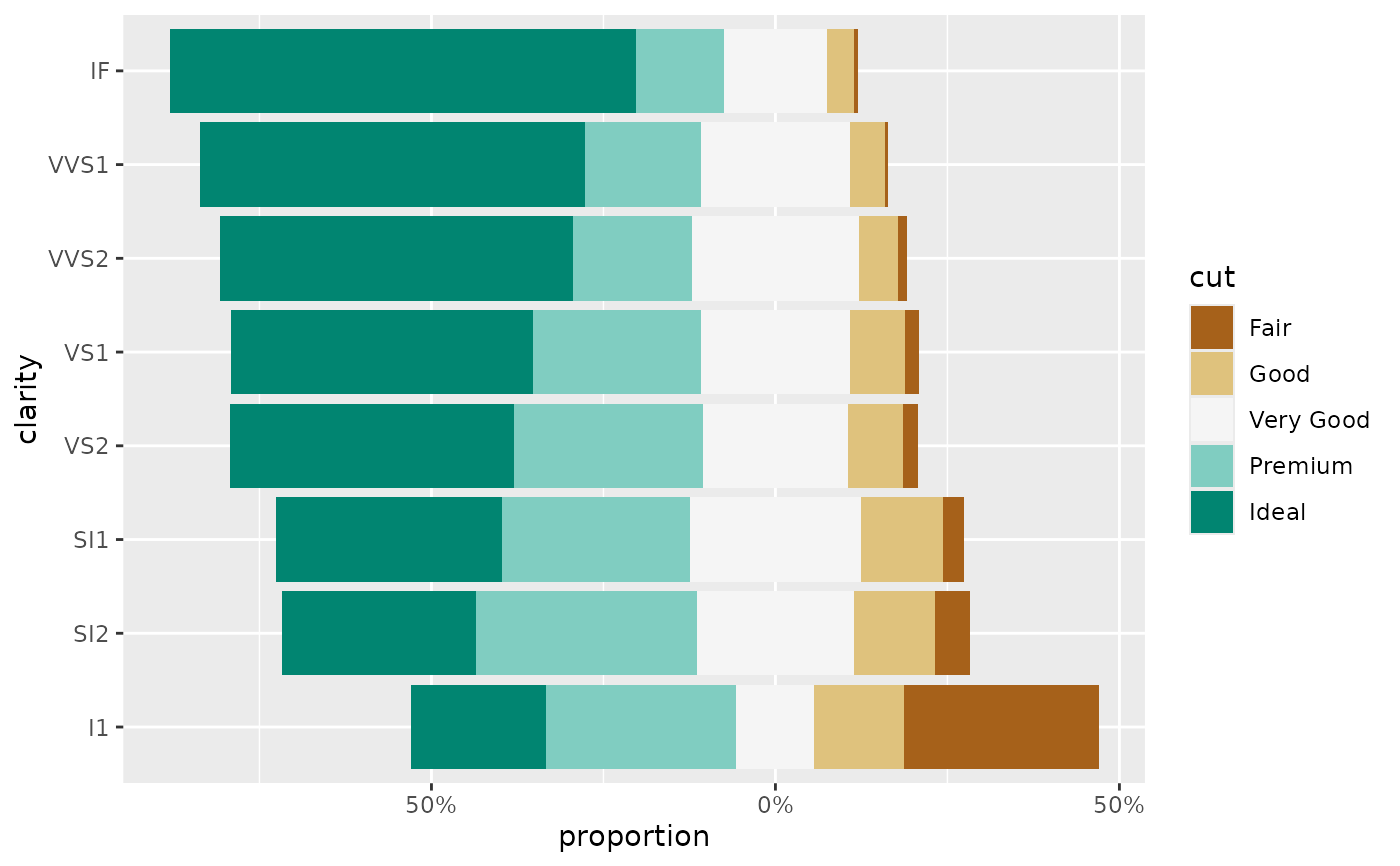# Missing items -------------------------------------------------------------
# example with a level not being observed for a specific value of y
d <- diamonds
d <- d[!(d$cut == "Premium" & d$clarity == "I1"), ]
d <- d[!(d$cut %in% c("Fair", "Good") & d$clarity == "SI2"), ]

# by default, the two lowest bar are not properly centered
ggplot(d) +
aes(y = clarity, fill = cut) +
geom_bar(position = "likert") +
scale_fill_brewer(palette = "PiYG")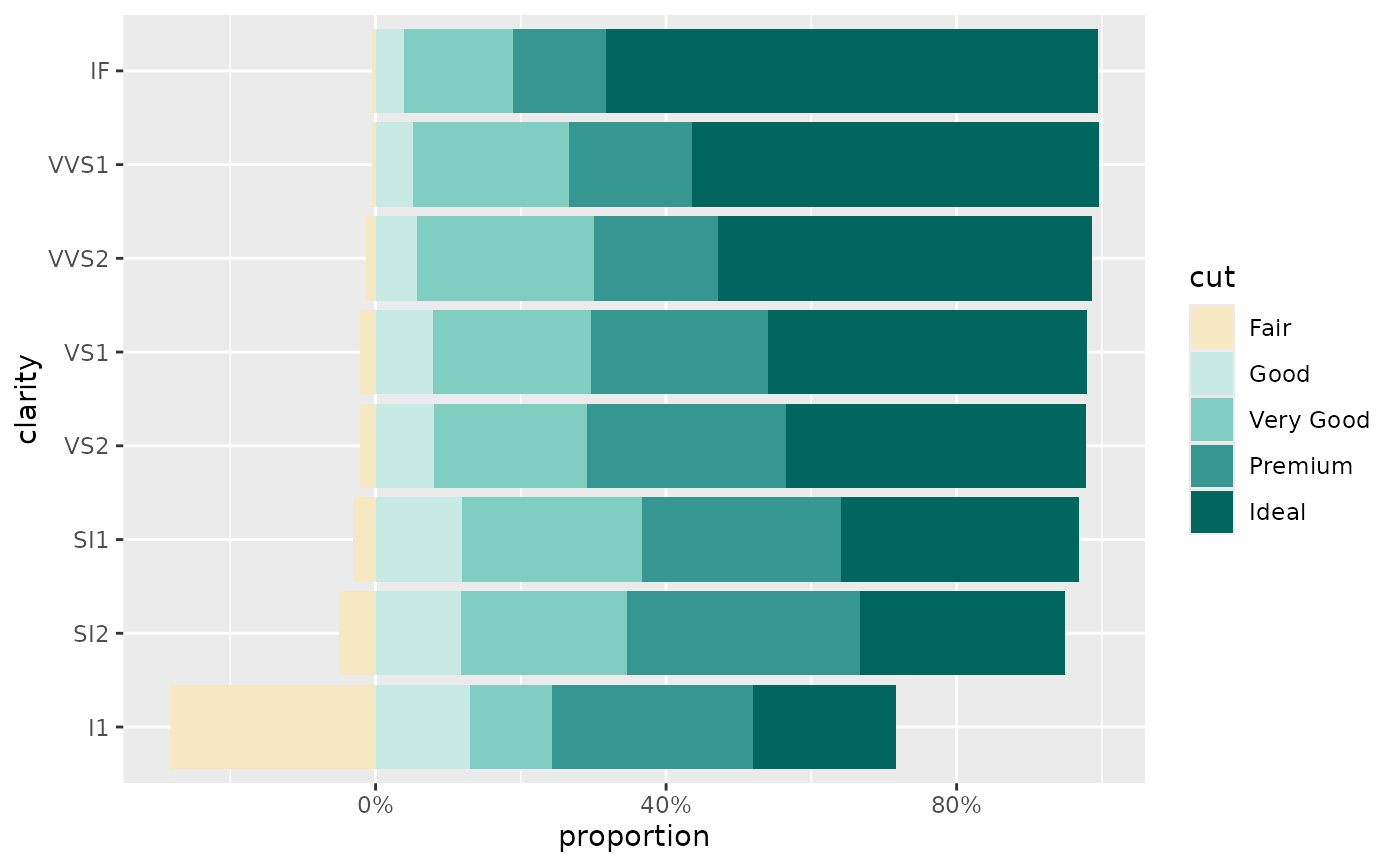# use stat_prop() with complete = "fill" to fix it
ggplot(d) +
aes(y = clarity, fill = cut) +
geom_bar(position = "likert", stat = "prop", complete = "fill") +
scale_fill_brewer(palette = "PiYG")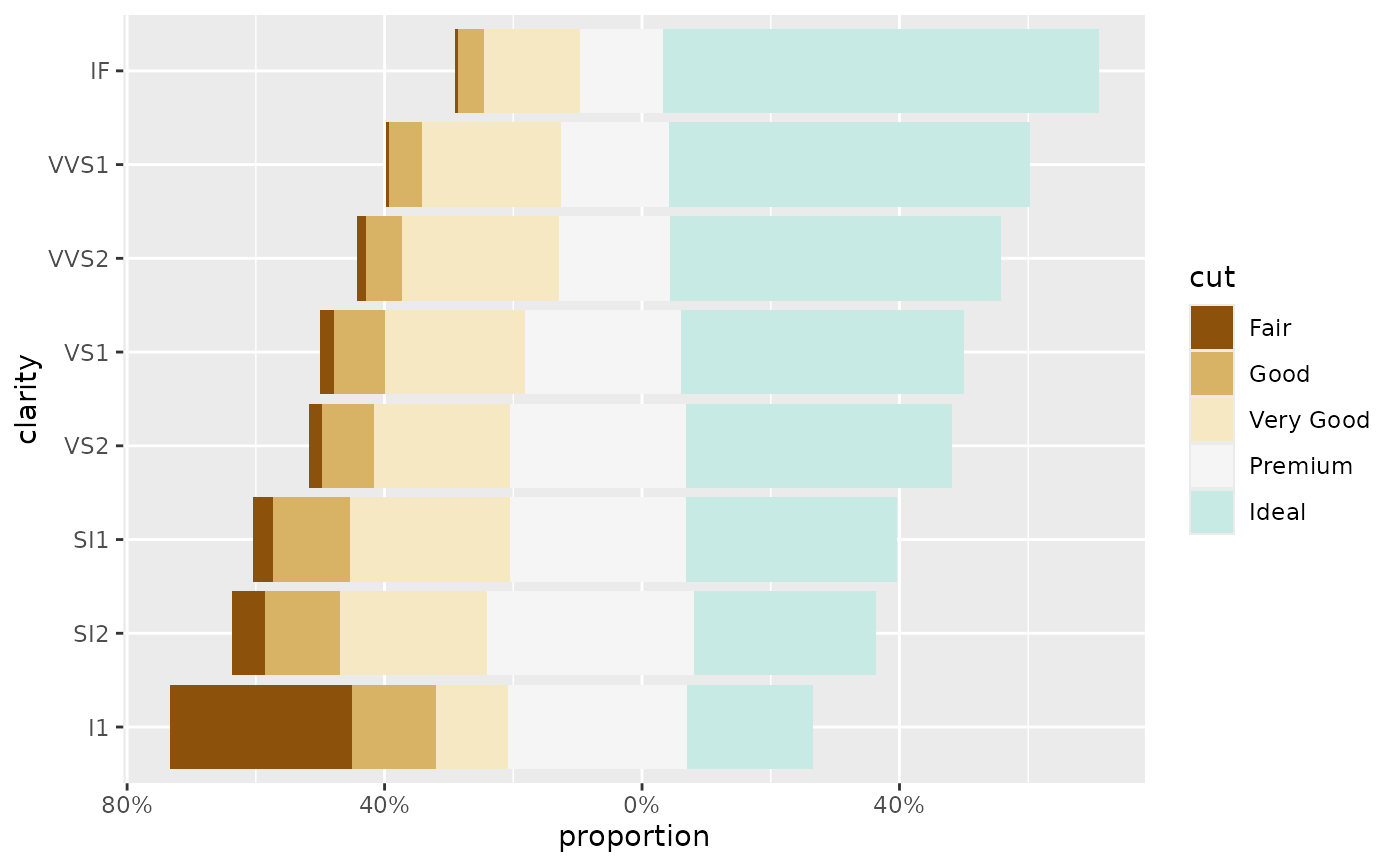custom_label <- function(x) {
p <- scales::percent(x, accuracy = 1)
p[x < .075] <- ""
p
}

ggplot(diamonds) +
aes(y = clarity, fill = cut) +
geom_bar(position = "likert") +
geom_text(
aes(by = clarity, label = custom_label(after_stat(prop))),
stat = "prop",
position = position_likert(vjust = .5)
) +
scale_x_continuous(label = label_percent_abs()) +
scale_fill_brewer(palette = "PiYG", direction = -1) +
xlab("proportion")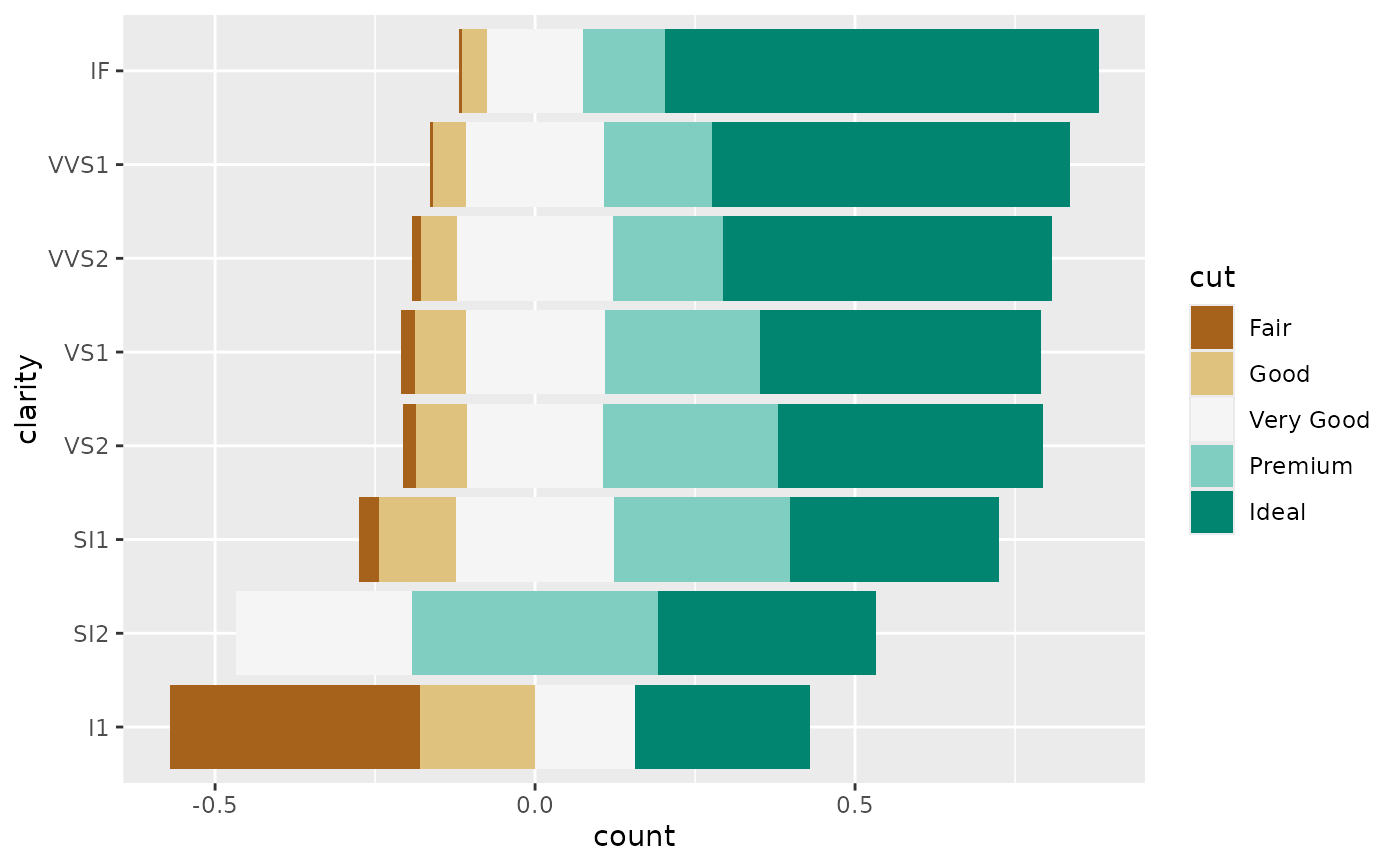# Do not display specific fill values ---------------------------------------
# (but taken into account to compute proportions)

ggplot(diamonds) +
aes(y = clarity, fill = cut) +
geom_bar(position = position_likert(exclude_fill_values = "Very Good")) +
scale_x_continuous(label = label_percent_abs()) +
scale_fill_brewer(palette = "PiYG") +
xlab("proportion")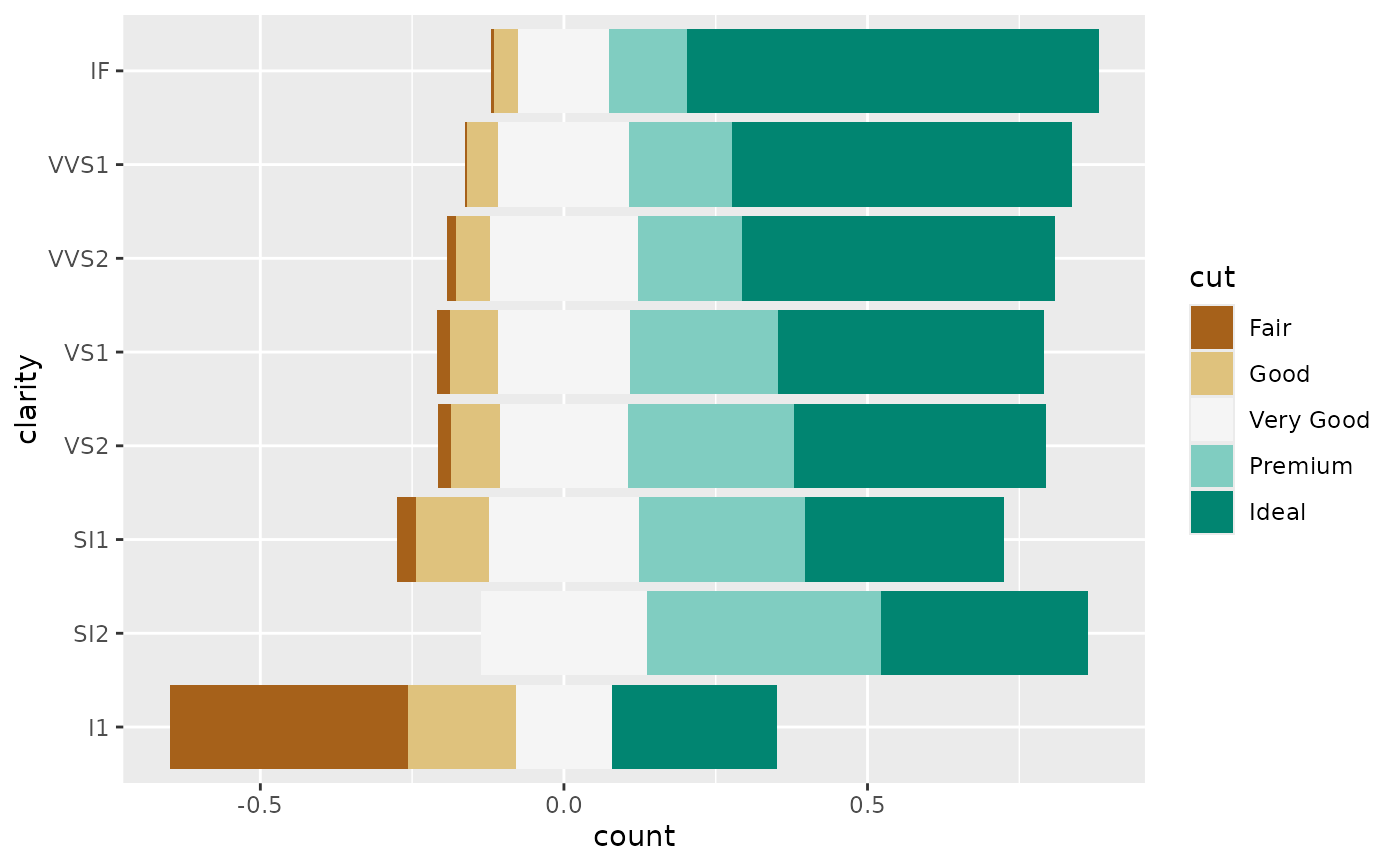# }